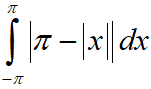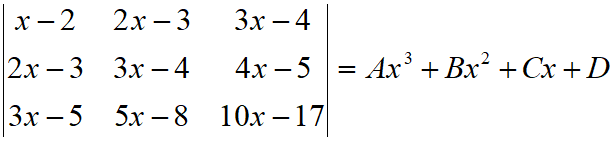Friday, October 22, 2021
Home > IIT JEE Main and Advance > JEE Main 2020 Question Paper Answer Keys Solutions 3 September Morning Shift

# JEE Main 2020 Question Paper Answer Keys Solutions 3 September Morning Shift

Hi Students, welcome to Amans Maths Blogs (AMB). On this post, you will get JEE Main 2020 Question Paper Answer Keys Solutions 3 September Morning Shift. As you know that Joint Entrance Examination means JEE Main and JEE Advanced is one of the most important entrance exam in engineering entrance examination. JEE Main 2020 is Computer Based Test (CBT) and it was conducted by the National Testing Agency (NTA). JEE Main 2020 exam duration is three hours and the exam consists of 300 marks (Maximum Marks). JEE Main 2020 Questions Paper has 2 sections in all three Subject Papers (Physics, Chemistry, Mathematics) and each subject has 25 questions.

## JEE Main 2020 Physics Questions Paper with Answer Keys & Solutions

In JEE Main 2020 Question Paper Answer Keys Solutions 3 September Morning Shift, Physics question paper’s answer keys and solutions by Resonance.

JEE Main 2020 Physics Question Paper with Answer Keys and Solutions (By Resonance)

## JEE Main 2020 Chemistry Questions Paper with Answer Keys & Solutions

In JEE Main 2020 Question Paper Answer Keys Solutions 3 September Morning Shift, Chemistry question paper’s answer keys and solutions by Resonance.

JEE Main 2020 Chemistry Question Paper with Answer Keys and Solutions (By Resonance)

## JEE Main 2020 Mathematics Questions Paper with Answer Keys & Solutions

In JEE Main 2020 Question Paper Answer Keys Solutions 3 September Morning Shift, Mathematics question paper’s answer keys and solutions by Amans Maths Blogs (AMB).

Instructions for SECTION 1 : (Maximum Marks : 80):

This section contains 20 multiple choice questions. Each question has 4 choices (1), (2), (3) and (4) for its answer, out of which Only One is correct.

JEE Main 2020 Maths Questions Answer Keys Solutions 3rd Sep Shift 1 : Ques No 1

Area enclosed by 0 ≤ y ≤ x2 + 1, 0 ≤ y x + 1, 1/2 ≤ x ≤ 2 is

Options:

A. 1/12

B. 1/6

C. 1

D. 1/3

Solution:

JEE Main 2020 Mathematics Questions with Answer Keys and Solutions : Ques No 2

If T1, T2, T3, … are in AP such that T1 + T2 + T3 + … + T25 = T26 + T27 + T28 + … + T40 and the first term is 3 then the values of common difference of AP is

Options:

A. 1/2

B. 1/6

C. 2

D. 3

Solution:

JEE Main 2020 Maths Questions Answer Keys Solutions 3rd Sep Shift 1 : Ques No 3, m, n ∈ HCF og (m, n) for least m & n.

Options:

A. 4

B. 3

C. 6

D. 9

Solution:

JEE Main 2020 Mathematics Questions with Answer Keys and Solutions : Ques No 4

A pair of dice is thrown and sum of dice come up multiple of 4 then find probability that at least one dice shows 4.

Options:

A. 2/7

B. 4/9

C. 1/9

D. 5/8

Solution:

JEE Main 2020 Maths Questions Answer Keys Solutions 3rd Sep Shift 1 : Ques No 5

Letbe a 2 x 2 matrix such that A4 = [aij]2×2a11 = 109, then find a22.

Options:

A. 12

B. 4

C. -8

D. 10

Solution:

JEE Main 2020 Mathematics Questions with Answer Keys and Solutions : Ques No 6

The equation of curve satisfying equation (1 + y2)(ex + 1)dy = exy2 and also passes through the point (0, 1) is

Options:

A. y2 + 1 = yln[(1 + ex) / 2]

B. y2 – 1 = yln[(1 + ex) / 2]

C. y + 1 = yln[(1 + ex) / 2]

D. 2y2 + 1 = yln[(1 + ex) / 2]

Solution:

JEE Main 2020 Maths Questions Answer Keys Solutions 3rd Sep Shift 1 : Ques No 7

Let x2 / 4 + y2 / 3 = 1 is an ellipse and a hyperbola which confocal with ellipse such that its transverse excess is √2 then which of the following point does not lie on hyperbola.

Options:

A. (1, – 1/√2)

B. (-√(3/2), 1)

C. (√(3/2), 1/√2)

D. None of these

Solution:

JEE Main 2020 Mathematics Questions with Answer Keys and Solutions : Ques No 8

2π – [sin-1 4/5 + sin-1 5/13 + sin-1 16/]

Options:

A. π/2

B. π

C. 3π/2

D. -π/2

Solution:

JEE Main 2020 Maths Questions Answer Keys Solutions 3rd Sep Shift 1 : Ques No 9

If y2 + ln cos2x = y then

Options:

A. |y”(0)| = 2

B. |y'(0)| + |y”(0)| = 1

C. |y'(0)| + |y”(0)| = 3

D. None of these

Solution:

JEE Main 2020 Mathematics Questions with Answer Keys and Solutions : Ques No 10(finite) where [.] denotes the greatest integer function then find L.

Options:

A. 0

B. 1/2

C. 1

D. 2

Solution:

JEE Main 2020 Maths Questions Answer Keys Solutions 3rd Sep Shift 1 : Ques No 11

Let α and β are roots of x2 + px + 2 = 0 and 1/α and 1/β are roots of 2x2 – 2qx + 1 = 0. Then find the value of (α + 1/β)(β + 1/α)(α – 1/α)(β – 1/β).

Options:

A. 9(9 – p2)/4

B. 9(9 + p2)/4

C. 4(9 – q2)/9

D. 9(9 – q2)/4

Solution:

JEE Main 2020 Mathematics Questions with Answer Keys and Solutions : Ques No 12

Evaluate.

Options:

A. π2

B. π2/2

C. π2/3

D. π2/4

Solution:

JEE Main 2020 Maths Questions Answer Keys Solutions 3rd Sep Shift 1 : Ques No 13Find k.

Solution:

JEE Main 2020 Mathematics Questions with Answer Keys and Solutions : Ques No 14

The value ofis.

Solution:

JEE Main 2020 Maths Questions Answer Keys Solutions 3rd Sep Shift 1 : Ques No 15

S = (2 1P0 – 3 2P1 + 4 3P2 + … to 51 terms) + (1! – 2! + 3! – 4! + … to 51 terms), then find S

Options:

A. 1 + 51!

B. 1 + 52!

C. 1 + 50(51!)

D. 1 + 51(51!)

Solution:

JEE Main 2020 Mathematics Questions with Answer Keys and Solutions : Ques No 16

The preposition p ⇒ (~(p ⋀ ~q)) is equivalent to

Options:

A. q

B. ~p V q

C. p V ~q

D. ~p V ~q

Solution:

JEE Main 2020 Maths Questions Answer Keys Solutions 3rd Sep Shift 1 : Ques No 17

The center of circle lies on x + y = 3 and touching the lines x = 3 and y = 3 then find the diamter of circle.

Solution:

JEE Main 2020 Mathematics Questions with Answer Keys and Solutions : Ques No 18

(21/2 + 51/8) has 33 integrals terms find least value of n is

Options:

A. 256

B. 257

C. 258

D. 259

Solution:

JEE Main 2020 Maths Questions Answer Keys Solutions 3rd Sep Shift 1 : Ques No 19

The area in sq unit of the region {(x, y); 1/2 ≤ y ≤ sin x, 0 ≤ x ≤ π} is equal to

Options:

A. 3 – 2π

B. √3 – π/6

C. 3 – π/3

D. √3 – π/3

Solution:

JEE Main 2020 Mathematics Questions with Answer Keys and Solutions : Ques No 20

Let the data 4, 10, x, y, 27 be in increasing order. If the median of data is 18 and its mean deviation about mean is 7.6 then mean of this data is

Options:

A. 17

B. 16

C. 16.5

D. 15.5

Solution:

JEE Main 2020 Maths Questions Answer Keys Solutions 3rd Sep Shift 1 : Ques No 21

A bag contains 6 red and 10 green balls, 3 balls are drawn from it one by one without replacement. If the third ball drawn is red, then the probability, that first two balls are green is

Options:

A. 3/7

B. 9/149

C. 9/56

D. 3/8

Solution:

JEE Main 2020 Mathematics Questions with Answer Keys and Solutions : Ques No 22

If, then find the absolute value of B + C